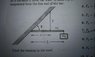# Torque on a suspended uniform bar

• nautola

## Homework Statement

A bar of length L supports a mass, m2 at the end. It is fastened by a pivot at one end to a wall which is at an angle θ to the horizontal. The bar is supported by a cord a distance x from the wall. The mass of the uniform bar is m1. I already solved for the tension: T=(1/2m1 + m2) (L/x) g

What is the horizontal component of the force exerted on the bar by the wall? Let right be the positive direction.

τ= Ʃ(Fdsinθ) τ=0

## The Attempt at a Solution

I set the torques equal to each other at the end with the mass, m2 so that I could determine Ncosθ, the vertical component of the normal force exerted by the wall. I also tried using the same torque equations at the midpoint of L and where T is exerted.

#### Attachments

•IMAG0032.jpg
25.2 KB · Views: 838
I assume you solved for T by looking at a free body diagram of the bar and summing torques about the left end of the bar. You migbt want to look at that same FBD to solve for the horizontal force component of the wall on the bar by using Newton's first law in the horizontal direction.

Is the answer x=0? Because it seems to me that the bar is pushing straight up on the wall, so that just makes the wall push straight down and be done.

My main confusion comes from the free body diagram that I'm looking at. I keep setting it up and I'm not sure if the forces should go perpendicular to the wall, or if they should go straight. Assuming they go perpendicular, I'm not entirely sure how to solve for the horizontal component.

Free Body Diagrams tell no lies. You seem to be questioning it,which is fine. But the sum of forces for a body in equilibrium must always be 0. If there is a horizontal force of the wall on the bar, there must be another horizontal force on the bar balancing it, but where ?

Is the answer x=0? Because it seems to me that the bar is pushing straight up on the wall, so that just makes the wall push straight down and be done.
Yes
My main confusion comes from the free body diagram that I'm looking at. I keep setting it up and I'm not sure if the forces should go perpendicular to the wall, or if they should go straight. Assuming they go perpendicular, I'm not entirely sure how to solve for the horizontal component.
You can break up the vert force of the wall on the bar into components perpendicular (normal) and parallel to the wall if you want. But their resultant must be in a vert direction.:zzz: